RADanalysis package has tools for normalizing rank abundance distributions (RAD) to a desired number of ranks using MaxRank Normalization method1. RADs are commonly used in biology/ecology and mathematically equivalent to complementary cumulative distributions (CCDFs) which are used in physics, linguistics and sociology and more generally in data science. For using the package you should first install and load the package.

#install.packages("RADanalysis")
library(RADanalysis)

Rank Abundance Distributions (RADs) are a way to capture the distribution of biological species in communities, where we use the term “species” for all types of distinct biological entities, e.g. microbial species in a microbiome, viral strains in a quasi-species, the diverse variants B cells in a person, etc. A RAD can be thought of as a plot with the two axes rank (x-axis) and abundance (y-axis). For the most abundant species we draw a point at the (x,y) coordinates $$(1,a1)$$, with $$a1$$ the abundance of this most abundant species. For the second most abundant species we draw a point at $$(2,a2)$$.

This is a first 10 rows of a typical RAD:

rad <- data.frame(rank = 1:100, abundance = round(1./(1:100) * 100))
knitr::kable(head(rad, 10))
rank abundance
1 100
2 50
3 33
4 25
5 20
6 17
7 14
8 12
9 11
10 10
plot(rad,xlab = "Rank",ylab = "Abundance",pch = 19,type = "b",lwd = 0.5)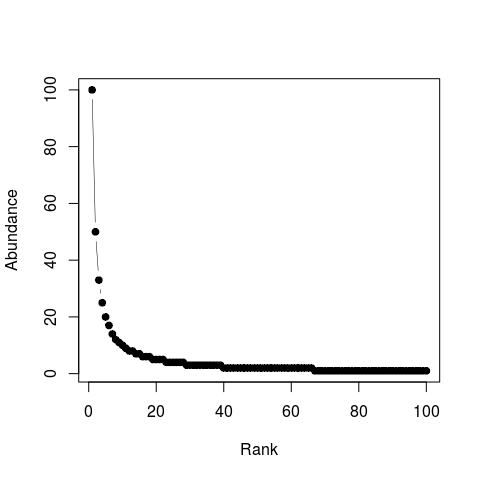A typical Rank Abundance Distribution in linear-linear scale

As is typical for a biological community, the RAD curve has a hollow shape. This means that the community has a small number of very abundant species, and a large number of rare species. The RADs may differ between communities, and that makes it interesting to compare them in order to learn about the communities. Since all RADs have such a hollow shape with a very long tail to the right, it is often more instructive to plot RADs in a log-log plot to see better major differences between RADs. This is the plot type we will be using in the following:

plot(rad,xlab = "Rank",ylab = "Abundance",pch = 19,log = "xy",type = "b",lwd = 0.5)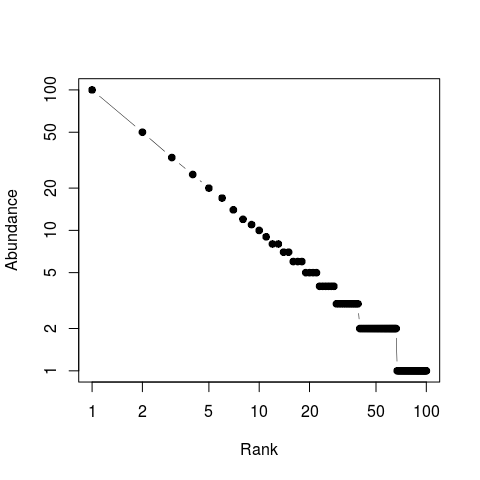A typical Rank Abundance Distribution in log-log scale

## RAD example: gut microbiome under treatment with antibiotic (Dethlefsen et al. 2008)

Dethlefsen et al. 2008 (http://journals.plos.org/plosbiology/article?id=10.1371/journal.pbio.0060280) have treated healthy individuals with the antibiotic Ciprofloxacin (Cp) and monitored the states of the gut microbiome before the treatment, during the treatment, and some time after the treatment. We will analyse their data using RADs.

In order to use the data you should first load the package. It is contained in the package as OTU table (OTU = operational taxonomic unit). In the following you can see the first $$10$$ columns of the data.

library(RADanalysis)
data("gut_otu_table")

colnames(gut_otu_table) <- c(paste("OTU_",seq(1:ncol(gut_otu_table)),sep = ""))
knitr::kable(gut_otu_table[,1:10])
OTU_1 OTU_2 OTU_3 OTU_4 OTU_5 OTU_6 OTU_7 OTU_8 OTU_9 OTU_10
A1 0 2 0 0 0 0 0 0 0 0
A2a 0 0 0 0 0 0 4 0 0 0
A2b 3 0 0 0 0 0 0 0 0 0
A2c 0 0 6 0 0 0 0 0 0 0
A3a 0 0 0 2 2 2 0 2 0 0
A3b 6 0 0 2 2 0 0 0 2 2
A4 0 0 0 0 0 0 0 0 0 0
A5 0 0 0 0 0 0 0 0 0 0
B1 0 0 0 0 0 0 0 0 0 0
B2 0 0 0 0 0 0 0 0 0 0
B3 0 0 0 0 0 0 0 0 0 0
B4 0 0 0 0 0 0 0 0 0 0
B5 0 0 0 1 0 0 0 0 0 0
C1 0 0 0 0 0 0 0 0 0 0
C2 0 0 0 0 0 1 0 0 0 0
C3 0 0 0 0 0 0 0 0 0 0
C4 0 0 0 0 0 0 0 0 0 0
C5 0 0 0 0 0 0 0 0 0 0

The OTU table contains abundances of all OTUs in all samples. Rows are samples, columns are OTUs. E.g. in sample A1, there was no observation of OTU 1 (abundance entry = 0), 2 observations of OTU 2, etc. “Observations” are here High-Throughput Sequencing (HTSeq) reads of 16S ribosomal genes. The sample names encode from which person the sample came (persons A, B, or C), and at which stage they were taken (1, 2: before treatment; 3: during treatment; 4, 5: after treatment). Thus, A1 is a sample of person A taken before the treatment.

### Create RADs form OTU table and plotting them

Making RADs from an OTU table means sorting the abundances decreasingly.

data("gut_otu_table")

line_cols <- c("green","red","blue")
#to specify different stages of subjects
sample_classes <- c(1,1,1,1,2,2,3,3,1,1,2,3,3,1,1,2,3,3)
plot(1,xlim = c(1,2000),ylim = c(1,20000),col = "white",log  = "xy",axes = F,
xlab = "Rank",ylab = "Abundance",main = "")
sfsmisc::eaxis(side = 1,at = c(1,10,100,1000))
sfsmisc::eaxis(side = 2)
temp <- temp[temp>0]
lines(x = temp,lwd = 2,col = line_cols[sample_classes[i]])
}
legend("bottomleft",bty = "n",legend = c("pre Cp","under Cp","post Cp"),
col = line_cols,lwd = 3)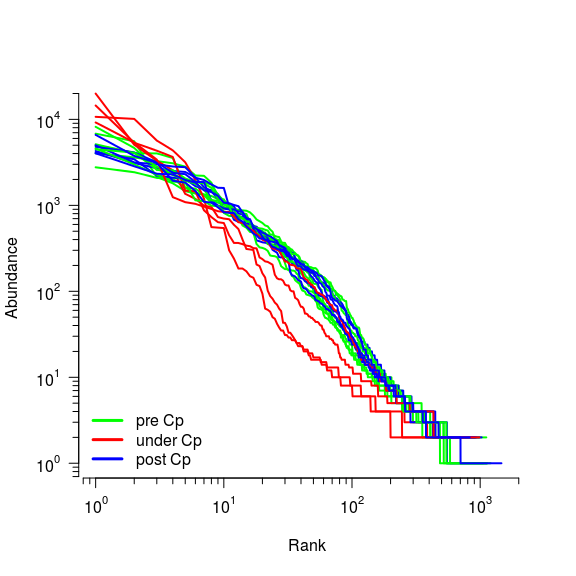Rank Abundance Distributions of gut microbiome data

### MaxRank normalization of the RADs

MaxRank normalization generates from a RAD with given richness $$S$$ a “normalized RAD” (NRAD) with a user-chosen smaller richness (MaxRank) $$R$$ . This is done by randomly re-sampling the original input of the RAD as long as the richness does not exceed $$R$$ . Since this is a random procedure, we repeat it $$n$$ times and average. MaxRank normalization allows us to turn several samples with different richness into a set of samples with the same richness. Mathematically, we turn vectors of different dimensions into vectors of the same dimension. It is easy to compare such vectors of the same dimension quantitatively. In the following we will normalize one of the RADs form gut_otu_table and will see the effect of averaging.

data(gut_otu_table)

#removing zeros
ylab = "Abundance", main = "",pch = 19,type = "b",cex = 0.5)

points(x = norm_rad$norm_rad * sum(norm_rad$norm_rad_count[1,]) ,pch = 19,cex = 1, type = "l",
col = "blue",lwd = 4)
points(x = norm_rad$norm_rad_count[1,],pch = 19,cex = 1, type = "l",col = "red",lwd = 3) points(x = norm_rad$norm_rad_count[2,],pch = 19,cex = 1, type = "l",col = "green",lwd = 3)
paste("nrad averaged over 50 realizations, times",
sum(norm_rad$norm_rad_count[1,]))), col = c("black","red","green","blue"),lwd = 2,bty = "n")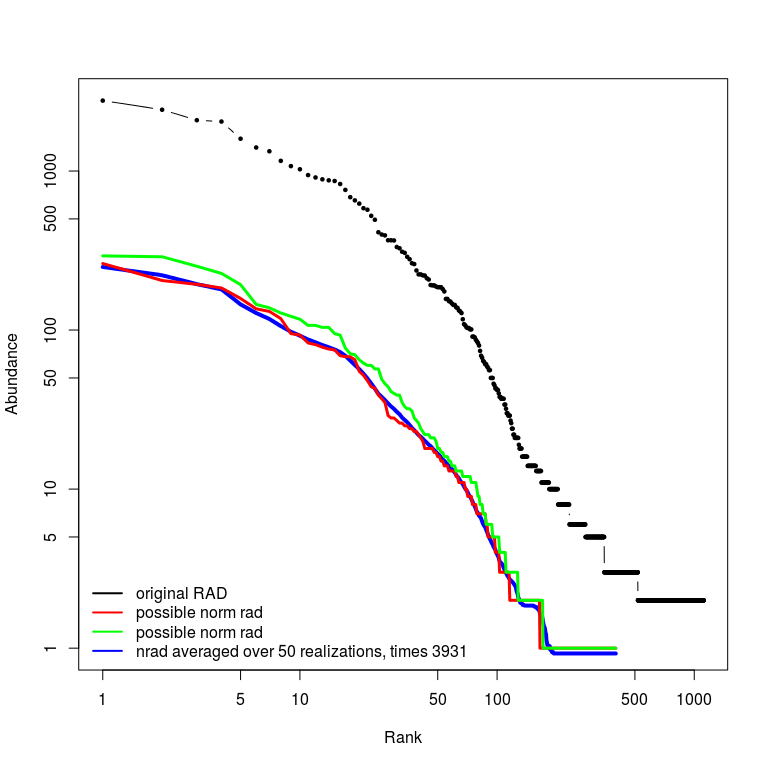Normalizing RADs using MaxRank normalization method with RADnormalization() In this example the first sample from gut_otu_table has been used. The original RAD has $$1116$$ ranks which is the total number of entries in the corresponding otu_table row which have positive numbers. In red and green you can see two of possible NRADs with $$R = 400$$. The blue curve shows the average over 50 possible NRADs. In the following we will work on NRADs that have been created by averaging. With the function RADnormalization() you can access all the NRADs used for averaging. In case you want to normalize a complete OTU table we recommend to use RADnormalization_matrix(). This function returns less data compared to RADnormalization() because sometimes the OTU tables are very big. ### MaxRank normalization of complete OTU table Let us generate from the RADs, the corresponding NRADs. We should first decide about $$R$$. To do so, we first look at the distribution of richness in our data set. data("gut_otu_table") rads <- gut_otu_table richness <- sapply(X = 1:nrow(rads), function(i) length(which(rads[i,] > 0))) boxplot(richness,horizontal = T,xlab = "Richness") quantile(richness) ## 0% 25% 50% 75% 100% ## 492.00 848.00 984.50 1122.75 1465.00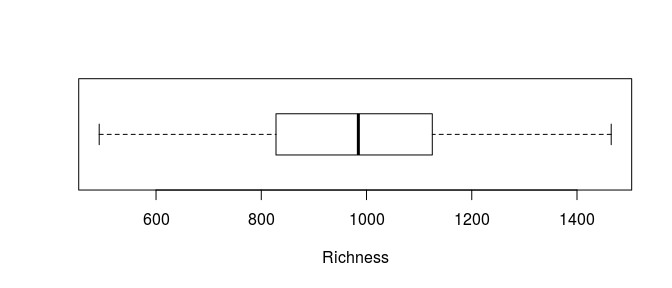Richness distribution in gut_otu_table So the richness (total number of ranks of each sample) is between $$492$$ and $$1465$$. In case richness is distributed normally, one can use the minimum richness for normalizing the complete OTU table. In case there are some outliers with very low richness we recommend to remove them from data set so that you could normalize table with higher $$R$$ and therefore lose less information. In this example I will use $$R=400$$ for simplicity. data("gut_otu_table") rads <- gut_otu_table #plot original rads line_cols <- c("green","red","blue") sample_classes <- c(1,1,1,1,2,2,3,3,1,1,2,3,3,1,1,2,3,3) #Normalization nrads <- RADnormalization_matrix(input = rads,max_rank = 400,average_over = 10, sample_in_row = TRUE,verbose = FALSE) nrads <- nrads$norm_matrix

plot(1,xlim = c(1,400),ylim = c(4e-5,1),col = "white",log  = "xy",
xlab = "Rank",ylab = "Abundance",
main = "")
lines(x = nrads[i,],lwd = 2,col = line_cols[sample_classes[i]])
}
legend("bottomleft",bty = "n",legend = c("pre Cp","under Cp","post Cp"),
col = line_cols,lwd = 3)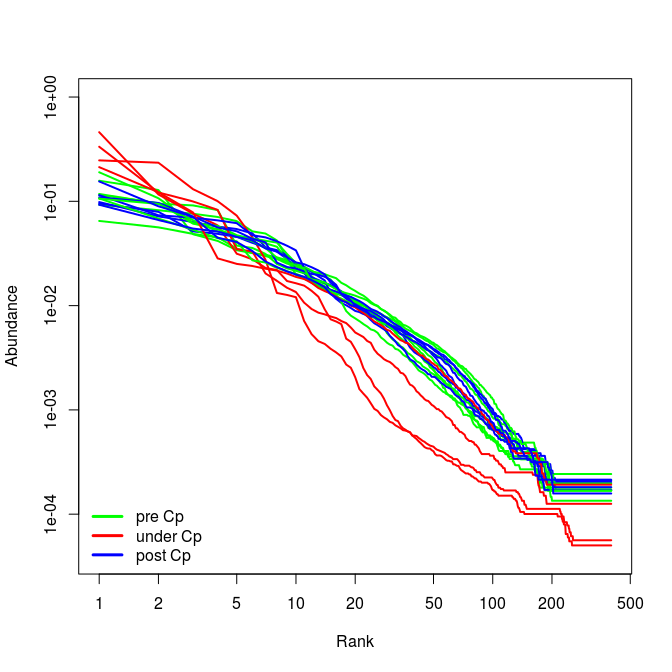NRADs of gut_otu_table with $$R=400$$ and averaged over 10 possible NRADs

Note that NRADs are treated as probability distributions, so they sum up to one. For the next examples I will use gut_nrads data which is included in the package. In gut_nrads I stored the result of RADnormalization_matrix(input = rads,max_rank = 400,average_over = 2000) to avoid computing them again and save a couple of minutes.

Now that we have NRADs we can continue processing them with ordination, clustering or classification methods. Here we only study an ordination example.

If the data consists of several groups, it is usually helpful to look at the averaged NRAD of each group. We call this averaged NRAD the $$"representative NRAD"$$. Here you can see the representative RADs for three groups of pre, under and post CP in the gut_otu_table.

data("gut_nrads")
nrads <- nrads$norm_matrix line_cols <- c("green","red","blue") sample_classes <- c(1,1,1,1,2,2,3,3,1,1,2,3,3,1,1,2,3,3) maxrank <- 400 #plot nrads plot(1e10,xlim = c(1,maxrank),ylim = c(2e-5,1),log="xy", xlab = "rank",ylab = "abundance",cex.lab = 1.5,axes = FALSE) sfsmisc::eaxis(side = 1,at = c(1,10,100,1000,10000)) sfsmisc::eaxis(side = 2,at = c(1e-4,1e-3,1e-2,1e-1,1),las = 0) for(i in 1:nrow(nrads)){ points(nrads[i,],type = 'l',col = line_cols[sample_classes[i]],lwd = 0.8) } #plot confidence intervals of representative nrads a <- representative_RAD(norm_rad = nrads,sample_ids = which(sample_classes == 1), plot = TRUE,confidence = 0.9,with_conf = TRUE, col = scales::alpha(line_cols,0.5),border = NA) a <- representative_RAD(norm_rad = nrads,sample_ids = which(sample_classes == 2), plot = TRUE,confidence = 0.9,with_conf = TRUE, col = scales::alpha(line_cols,0.5),border = NA) a <- representative_RAD(norm_rad = nrads,sample_ids = which(sample_classes == 3), plot = TRUE,confidence = 0.9,with_conf = TRUE, col = scales::alpha(line_cols,0.5),border = NA) #plot representative nrads a <- representative_RAD(norm_rad = nrads,sample_ids = which(sample_classes == 1), plot = TRUE,with_conf = FALSE, col = scales::alpha(line_cols,0.8),lwd = 4) a <- representative_RAD(norm_rad = nrads,sample_ids = which(sample_classes == 2), plot = TRUE,with_conf = FALSE, col = scales::alpha(line_cols,0.8),lwd = 4) a <- representative_RAD(norm_rad = nrads,sample_ids = which(sample_classes == 3), plot = TRUE,with_conf = FALSE, col = scales::alpha(line_cols,0.8),lwd = 4) legend("bottomleft",bty = "n",legend = c("pre Cp","under Cp","post Cp"), col = line_cols,lwd = 3)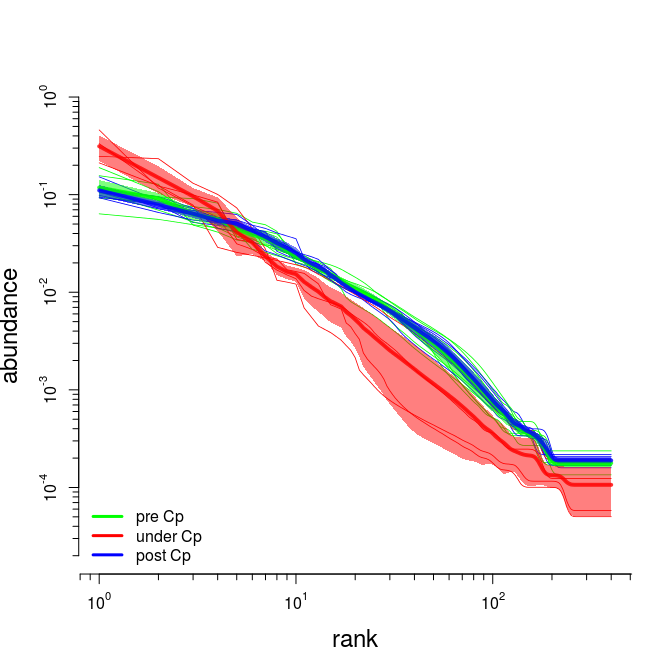Representative NRADs of three states of samples ## Ordination and representative points When there are more than $$10-20$$ samples, it is hard to recognize a structure in the NRADs. In this case it is usually helpful to use ordination methods such as multi-dimensional scaling. But before doing any ordination, we need to define a distance between NRADs. In the following example, we will use the $$Manhattan$$ distance between NRADs. You can also plot the representative points (mean of all the points in the group) to see better if there is a significant difference between groups. data("gut_nrads") nrads <- gut_nrads nrads <- nrads$norm_matrix

line_cols <- c("green","red","blue")
sample_classes <- c(1,1,1,1,2,2,3,3,1,1,2,3,3,1,1,2,3,3)
maxrank <- 400

#distance matrix using manhattan distance
d <- dist(x = nrads,method = "manhattan")
#ordination using classical multi-dimensional scaling
mds <- cmdscale(d = d,k = 5,eig = TRUE)

#plot the points
plot(mds$points,xlab = "First coordinate",ylab = "Second coordinate",pch = 19, cex =1,col = line_cols[sample_classes], main = "MDS plot with representative points \n of each group and error bars") #add the representative points wit erorr bar to the previous plot a <- representative_point(input = mds$points,ids = which(sample_classes == 1),
col = scales::alpha(line_cols,0.5),
plot = TRUE,standard_error_mean = TRUE,pch = 19, cex = 4)
a <- representative_point(input = mds$points,ids = which(sample_classes == 2), col = scales::alpha(line_cols,0.5), plot = TRUE,standard_error_mean = TRUE,pch = 19, cex = 4) a <- representative_point(input = mds$points,ids = which(sample_classes == 3),
col = scales::alpha(line_cols,0.5),
plot = TRUE,standard_error_mean = TRUE,pch = 19, cex = 4)

legend("bottomleft",bty = "n",legend = c("pre Cp","under Cp","post Cp"),
col = line_cols,pch = 19)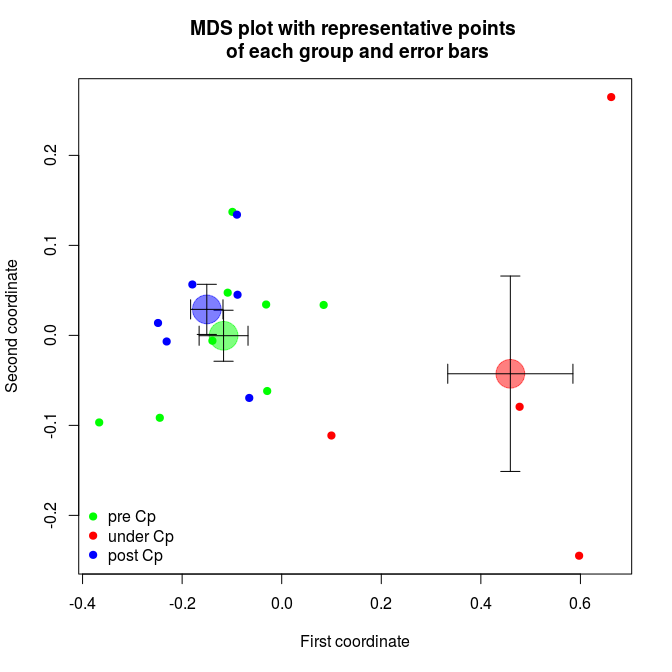Multi dimensional scaling of gut data using manhattan distance. Large points and errorbars are representative points and standard error of the mean for each group.

1. Saeedghalati et al. 2016 “Quantitative comparison of abundance structures of genetic communities”, submitted